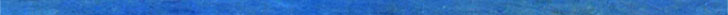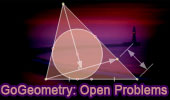# Geometry: Open Problems, High School, College, TutoringGeometry: Open Problems Geometry Problem 1494. Post a solutionParallelogram, Midpoints, Octagon, Areas. Dynamic Geometry 1480. Post a solutionJapanese Theorem for Cyclic Polygon, Sangaku,  Triangulation, Non-intersecting Diagonals, Sum of Inradii, Invariant, Step-by-step Illustration. GeoGebra, iPad. Dynamic Geometry 1475. Post a solutionClifford Intersecting Circles Theorem, Step-by-step Illustration, GeoGebra, iPad. Dynamic Geometry 1473. Post a solution Kosnita's Theorem, Triangle, Four Circumcenters, Concurrent Line, Step-by-step Illustration. Dynamic Geometry 1471. Post a solution Equilateral Triangle, Inside/Outside Point, Incenters, Tangency Points, Concurrent Lines, Step-by-step Illustration. Dynamic Geometry 1470. Post a solution Tangential Quadrilateral, Incircles, Tangent, Parallel, Rhombus, Step-by-step Illustration. Geometry Problem 1462. Post a solution Newton-Line, Newton-Gauss Line, Complete Quadrilateral, Midpoints of Sides and Diagonals, Collinear Points. Dynamic Geometry 1460. Post a solution Newton-Gauss Line, Complete Quadrilateral, Midpoints of Diagonals, Collinear Points, Step-by-step Illustration using GeoGebra. Geometry Problem 1458. Post a solution Triangle, Incircles, Excircle, Area, Step-by-step Illustration using GeoGebra. Dynamic Geometry 1451. Post a solution Orthopole of a Line. Step-by-step illustration using GeoGebra. Dynamic Geometry 1450. Post a solution Ortholine, Steiner Line, Complete Quadrilateral, Collineal Orthocenters. Step-by-step illustration using GeoGebra. Dynamic Geometry 1449. Post a solution Salmon Line. Step-by-step illustration using GeoGebra. Dynamic Geometry Problem 1444. Post a solution The Asymmetric Propeller Theorem, Equilateral Triangles, Midpoints. Using GeoGebra. Geometry Problem 1437. Post a solution Intersecting Circles, Common Tangent, Equal Product, Art, Poster, Typography, iPad Apps. Geometry Problem 1435. Post a solution Circle, Diameter, Inscribed Circles, Circular Sector, Parallelogram, Parallel Lines, Tangency Points, Poster, Typography, iPad Apps. Geometry Problem 1434. Post a solution Equilateral Triangle, Tangent Circles, Tangent Lines, Arithmetic Mean, Measurement, Poster, Typography, iPad Apps. Geometry Problem 1431. Post a solution Triangle, Circumcircle, Parallel Chord, Apollonius, LLC, Congruent Angles, Poster, Conformal Mapping. Geometry Problem 1417. Post a solution Triangle, Cevian, Circumcircle, Incenter, Perpendicular, Angle Bisector, Tangent Lines, Tangent Circles. Geometry Problem 1401. Post a solution Right Triangle with three circles on the sides, Isosceles, Diameter, Center, Tangent, Congruence. Geometry Problem 1396. Post a solution Triangle with three rectangles on the sides, Vertices, Perpendicular lines, Concurrency. Geometry Problem 1385. Post a solution Triangle, Orthocenter, Circle, Circumcircle, Angle Bisector, Midpoint, Collinear Points. Geometry Problem 1376. Post a solution Isosceles Triangle, Interior Cevian, Excircles, Tangency Points, Parallel Lines. Go to Page: Previous | 1 | 5 | Next

Home | Sitemap | Geometry | Problems | All Problems | Ten Geometry Problems | Visual IndexEmail
Last updated: Mar 9, 2022